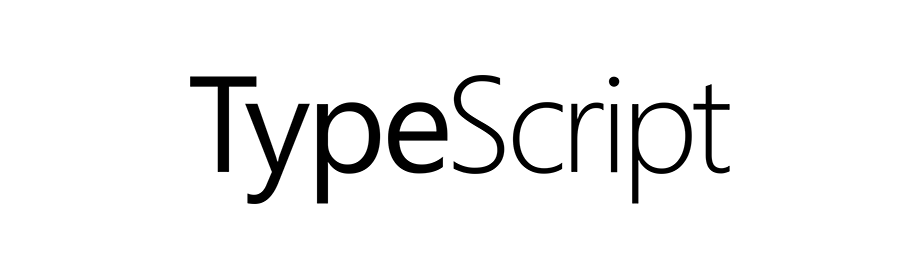# TypeScript - Arrow Functions

TypeScriptIn this tutorial we will learn about arrow functions in TypeScript.

## What is an Arrow Function?

An Arrow Function a.k.a. Fat Arrow Function, are concise way of writing a function expression.

In the following example we have two ways of writing a function in ES5 and ES6 style of coding.

``````// ES5
var getResult = function(username, points) {
return username + ' scored ' + points + ' points!';
};

// ES6
var getResult = (username: string, points: number): string => {
return `\${ username } scored \${ points } points!`;
}
``````

## Arrow function with no parameters and no return value

To create an arrow function with no parameters and no return value we use the given syntax.

``````() => {}
``````

In the following example we have a function `fun1` that takes no arguments and returns no value.

``````var fun1 = () => {
console.log("Hello World");
};

// calling
fun1();
``````

The above code is similar to the following.

``````var fun1 = function() {
console.log("Hello World");
};
``````

## Arrow function with no parameters but returns some value

Following is the syntax of an arrow function having no parameters but returns some value.

``````(): type => {
return expr;
}
``````

In the following example we have a function `fun2` that takes no argument but returns a value of type `number`.

``````var fun2 = (): number => {
return Math.random();
};

// calling
console.log("Random number: " + fun2());
``````

The above code is similar to the following code.

``````var fun2 = function() {
return Math.random();
};
``````

## Arrow function with parameters but no return value

Following is the syntax of an arrow function having some parameters but returns no value.

``````(v1: type, v2: type, ...): void => {}
``````

In the following example we have a function `fun3` that takes two arguments (string and number) and returns no value.

``````var fun3 = (username: string, points: number): void => {
let str = `\${ username } scored \${ points } points!`;
console.log(str);
};

// calling
fun3('Yusuf Shakeel', 10);
``````

The above code is similar to the following.

``````var fun3 = function(username, points) {
var str = username + ' scored ' + points + ' points!';
console.log(str);
};
``````

## Arrow function with parameters and returns some value

Following is the syntax of an arrow function having some parameters and returning some value.

``````(v1: type, v2: type, ...): type => {
return expr;
}
``````

In the following example we have a function `fun4` that takes two arguments (string and number) and returns a string value.

``````var fun4 = (username: string, points: number): string => {
return `\${ username } scored \${ points } points!`;
};

// calling
console.log(fun4('Yusuf Shakeel', 10));
``````

The above code is similar to the following.

``````var fun4 = function(username, points) {
return username + ' scored ' + points + ' points!';
};
``````